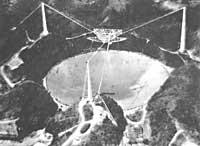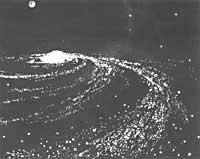The lost mass of the universe

1987/12/01 Arregi Bengoa, Jesus Iturria: Elhuyar aldizkaria

The theory of the Big Bang or the Standard Big Bang Model was a success when solving some problems about the origin and evolution of the Universe.The Arecibo radio telescope is the largest in the world. However, there are suspicions that there is more matter than can be seen and detected in the Universe through telescopes and radio telescopes.

This theory explains the expansion of the Universe discovered by Hubble as a result of an initial explosion. The law of gravity is the counterpoint of expansion, which works by reducing the speed of distance between all objects. Analysis of these adverse effects suggests a question of the future: Will the expansion of the universe remain indefinitely or will the influence of gravity paralyze expansion and cause another collapse similar to the time of its creation?

The answer has to do with the amount of materi of the Universe. The minimum value of density for gravity to stop expansion is called critical density and is expressed by c. Of course, the higher the speed of propagation, the greater the values necessary for critical density. Therefore, it is calculated from the measurements of the velocities of distance of galaxies c and the value currently admitted c = 2. 10 – 29 g/cm 3, that is, approximately 10 atoms of hydrogen per cubic meter.

If the value of the present density of the Universe was a critical value ( c ), we should assume that the Universe is infinite, its geometry being a typical euclidean geometry, that is, a flat geometry. In this case, the force of gravity would paralyze the propagation, but it could not cause the subsequent collapse. If the current density is greater than c, the Universe would be finite, spherical geometry — the shortest distance between two points would not be a straight, but a circle arc — and would follow the collapse of expansion.

If we would not achieve critical value 0, we would live in an infinite Universe and hyperbolic geometry. These options are usually indicated by parameter 0 = 0 / c. As is obvious, the first option, that of the plain universe, corresponds to case 0 = 1, that of the spherical to 0 1-i and that of the hyperbolic to 0 1-i. Therefore, it is very important to obtain reliable measurements of the density and in recent years the unforeseen events have occurred from the sessions carried out for it.

The suspicion that there is more matter than can be seen and detected in the universe through telescopes and radio telescopes is not the current one. J. Oort in 1930 and F. Zwicky” proposed in 1933 the need for an invisible matter to explain some movements of the system. However, what no one expected is that the matter we have been able to detect through its divination is only one hundredth part of the universe. However, current theories go that way. The existence of a large quantity of undetected masses generates problems about its nature, consolidating the hypothesis that matter can be non-varionic. That is, that emptiness that has become evident would not be that of protons, neutrons (bariones) and known total electrons, but other special fractions generated in the first steps of the evolution of the Universe.

The experimental bases that support these conclusions have been obtained at the level of galaxies and clusters of galaxies. On the other hand, at cosmological level, some calculations based on the theories that we consider well established reinforce the conclusions suggested by these measurements.

As for the experimental bases, the first test is provided by the measurements of the rotation movement of galaxies. According to them, the rotational velocity of hydrogen clouds located on the outside of the galaxy discs (up to 30 kiloparsec) is no less than that of the stars and clouds of the interior, as predicted by Newton's gravitational law. As can be seen in the figure, it is described by a curve that evolves at slow speed, forcing the existence of an influence that is supposedly manifested through an existing mass.

According to the values obtained, the mass of a common galaxy would multiply what we have detected thanks to its electromagnetic emission, that is, we do not perceive 90% of the mass of galaxies. If in the Universe there were only the mass that we could see, the value of the density would be 1% or 2% of the value necessary for the Universe to occur. Accepting the mass increase necessary to explain the rotation speed of galaxies, it would be between 10% and 20% of the critical value 0.

When analyzing the clusters and superclusters, the results are similar. Despite considering as a mass of galaxies the necessary to explain the speed of rotation, the dynamic analysis of these structures reveals that to explain the stability of the clusters and superclusters, which has become evident in other ways, more mass is needed than the contribution of galaxies.

In view of these results we can think that in the measurement process there is some systematic error, but it is a very important theoretical argument that affirms the reliability of the experimental results.

In the first pins of the evolution of the universe, the lighter elements emerged through nucleosynthesis processes. The quantities of the elements that were formed then depended, logically, on the number of protons and neutrons that existed in the place. Taking into account this, the hydrogen abundance of these present elements allows limiting the abundance of barions per hour. As this quantity is constant, you can calculate the density that generates currently. By putting numbers it is possible that the density of the common matter is placed around 20% of c.

With what has been said in the last paragraphs, and although the amount of mass necessary to explain the dynamics of galaxies and clusters is very large, an open and hyperbolic universe can be admissible, with an unvisible matter also common, but this hypothesis cannot be maintained within the panorama described by cosmological theories.

Year 1980 A. H. H. Guth, based on the new theories of fractions, added to the big-bang a profound improvement, explaining the problem of laicity among other successes. Today the Universe, to the limits that we know, is four, and we have evidence of what has so far been, but according to the big-bang model if the initial value of W had not been 1, would have distanced much from the value of the evolution of the Universe, making an evident curvature. According to the theory of the inflationary universe (often referred to as Guth's theory), the Universe raised 10 times its volume (so the reference to inflation) in a very early and brief phase of its evolution (t = 10 –35” first and 10 –32” duration).

This huge expansion made the curvature of the Universe disappear almost completely, as it decreases when we inflate a globe. Therefore, the value of became practically one and the change that has suffered since then is not enough to be evident. We must therefore recognize that 0 = 1, that is, 0 = c, that the universe is flat and that, therefore, at least 80% of the present matter is not formed by protons and neutrons.Our galaxy, the Milky Way. The measurement of the rotation movement of galaxies can help solve the problem of the hidden mass of the Universe.

It's time to directly address the topic we already mentioned in the title, responding to two main questions. One on the distribution of this cold matter and another, of course, on its nature.

As for the first, researchers have seriously considered the option that galaxies are not representative of the major condensations of matter. According to the data we have given above, the mass of galaxies only accounts for 20% of c. Therefore, 80% enough for the Universe to occur would be in regions where there are no galaxies.

On nature we cannot say too many concrete things. The problem is quite unknown for there to be many candidates. Perhaps what can be affirmed with greater certainty is the inadequacy of the aforementioned candidates (neutrinos). The main reason for the exclusion is that, after forming superclusters and clusters in a universe full of neutrinos, the galaxy would take a long time to condense, ending the process with half the age of the Universe. The existence of ancient structures such as the coarse denies the validity of the hypothesis.

All other candidates present an important initial error: that their existence be merely theoretical. Moreover, it seems that some of them could be sufficient to occupy the place of unperceived cold matter. Most are needs of the physics theories of fractions and can be classified into two large groups: the light bosons, that is, the light fractions of the entire spine (between 10 – 14 and 10 – 10 of its protocol mass) and the heavy fractions (of the intermediate mass between 1 and 1000 of the proton).

The fraction of the first group that has been given more options is axio. This fraction is a necessity created by an existing symmetry between the main interactions in high energy levels. These axions, by breaking the symmetry, would create a background area (similar to the background microwave radiation) for their subsequent accumulation in large groups, explaining the lost matter. Among those of the second group, the most prestigious are those derived from the theory of supersymmetry. Supersymmetry is a new symmetry that relates fermions (half-spine fractions, for example, protons neutrons and electrons) and bosons (full spine, such as photon and gluon).

This would unify the four known major interactions (electromagnetic, weak, violent and gravity) in one and predict a new one for each known fraction so far: photin, gravitin, electron, selectron, neutrino, sneutrino, etc. Among all of them, perhaps the gravitino and the fotino stand out. Gravity, against the case of neutrino, can explain the formation of galaxies, but not that of superclusters. Photins with an intermediate mass between periods 1 and 50 of the mass of the proton could also form part of the lost mass, but for them there are also unovercome difficulties.

Finally, mention two new candidates: magnetic monopoles and big-bang strings. These are not fractions, but topological errors produced in the first steps of the evolution of the Universe. But lately much has been spoken of these creatures. Therefore, in the following number we will analyze more in depth.eu es fr en ca gl

Gai honi buruzko eduki gehiago

Elhuyarrek garatutako teknologia

1989-9-1Zientzia
1988-2-1Zientzia